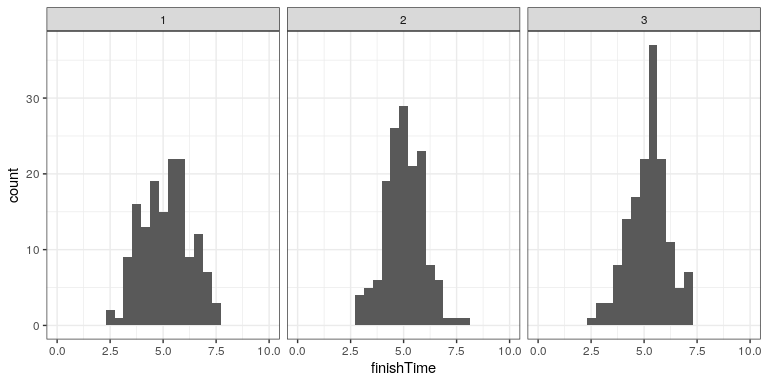# 14.4: Using Monte Carlo Simulation

$$\newcommand{\vecs}{\overset { \rightharpoonup} {\mathbf{#1}} }$$ $$\newcommand{\vecd}{\overset{-\!-\!\rightharpoonup}{\vphantom{a}\smash {#1}}}$$$$\newcommand{\id}{\mathrm{id}}$$ $$\newcommand{\Span}{\mathrm{span}}$$ $$\newcommand{\kernel}{\mathrm{null}\,}$$ $$\newcommand{\range}{\mathrm{range}\,}$$ $$\newcommand{\RealPart}{\mathrm{Re}}$$ $$\newcommand{\ImaginaryPart}{\mathrm{Im}}$$ $$\newcommand{\Argument}{\mathrm{Arg}}$$ $$\newcommand{\norm}{\| #1 \|}$$ $$\newcommand{\inner}{\langle #1, #2 \rangle}$$ $$\newcommand{\Span}{\mathrm{span}}$$ $$\newcommand{\id}{\mathrm{id}}$$ $$\newcommand{\Span}{\mathrm{span}}$$ $$\newcommand{\kernel}{\mathrm{null}\,}$$ $$\newcommand{\range}{\mathrm{range}\,}$$ $$\newcommand{\RealPart}{\mathrm{Re}}$$ $$\newcommand{\ImaginaryPart}{\mathrm{Im}}$$ $$\newcommand{\Argument}{\mathrm{Arg}}$$ $$\newcommand{\norm}{\| #1 \|}$$ $$\newcommand{\inner}{\langle #1, #2 \rangle}$$ $$\newcommand{\Span}{\mathrm{span}}$$$$\newcommand{\AA}{\unicode[.8,0]{x212B}}$$

Let’s go back to our example of exam finishing times. Let’s say that I administer three quizzes and record the finishing times for each student for each exam, which might look like the distributions presented in Figure 14.2.Figure 14.2: Simulated finishing time distributions.

However, what we really want to know is not what the distribution of finishing times looks like, but rather what the distribution of the longest finishing time for each quiz looks like. To do this, we can simulate the finishing time for a quiz, using the assumption that the finishing times are distributed normally, as stated above; for each of these simulated quizzes, we then record the longest finishing time. We repeat this simulation a large number of times (5000 should be enough) and record the distribution of finishing times, which is shown in Figure 14.3.Figure 14.3: Distribution of maximum finishing times across simulations.

This shows that the 99th percentile of the finishing time distribution falls at 8.81, meaning that if we were to give that much time for the quiz, then everyone should finish 99% of the time. It’s always important to remember that our assumptions matter – if they are wrong, then the results of the simulation are useless. In this case, we assumed that the finishing time distribution was normally distributed with a particular mean and standard deviation; if these assumptions are incorrect (and they almost certainly are), then the true answer could be very different.

This page titled 14.4: Using Monte Carlo Simulation is shared under a not declared license and was authored, remixed, and/or curated by Russell A. Poldrack via source content that was edited to the style and standards of the LibreTexts platform; a detailed edit history is available upon request.